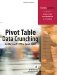# Sum, Average, Count, Min, Max, and More

 So far, every example in this chapter has involved summing data. It is also possible to get an average, minimum, or maximum of data. In VBA, change the Function property of the data field and give the data field a unique name. For example, the following code fragment produces five different summaries of the quantity field, each with a unique name: ` ' Set up the data fields With PT.PivotFields("Revenue") .Orientation = xlDataField .Function = xlSum .Position = 1 .NumberFormat = "#,##0,K" .Name = "Total Revenue" End With With PT.PivotFields("Revenue") .Orientation = xlDataField .Function = xlCount .Position = 2 .NumberFormat = "#,##0" .Name = "Number Orders" End With With PT.PivotFields("Revenue") .Orientation = xlDataField .Function = xlAverage .Position = 3 .NumberFormat = "#,##0" .Name = "Average Revenue" End With With PT.PivotFields("Revenue") .Orientation = xlDataField .Function = xlMin .Position = 4 .NumberFormat = "#,##0" .Name = "Smallest Order" End With With PT.PivotFields("Revenue") .Orientation = xlDataField .Function = xlMax .Position = 5 .NumberFormat = "#,##0" .Name = "Largest Order" End With ` The resultant pivot table provides a number of statistics about the average revenue, largest order, smallest order, and so on.Pivot Table Data Crunching for Microsoft Office Excel 2007
ISBN: 0789736012
EAN: 2147483647
Year: 2003
Pages: 140

Similar book on Amazon